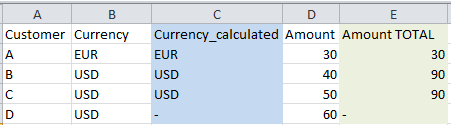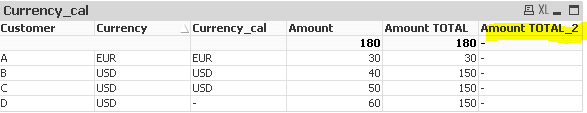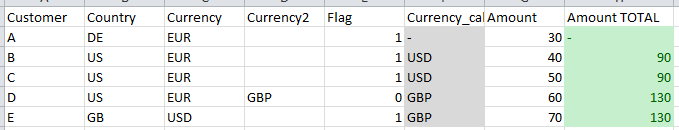QlikView App Development

Discussion Board for collaboration related to QlikView App Development.

Announcements
QlikWorld 2020: Join us May 11 - 14, 2020 in Phoenix, AZ. Register early and save \$400. Learn More
Highlighted
New Contributor III

SUM TOTAL by expression values

Hello,

is it possible to sum up values by the output of another expression using the TOTAL qualifier? Example:Column C is an calculated expression based on column B. I would like to aggregate the amount (column D) by the output of column C. The desired output is displayed in column E. In Qlikview, I am trying to achieve this result using the function TOTAL:However, I receive the following error: "Total specifier must be a valid field name" .Is there another way to solve this problem? Please find attached the qvw containing the example.

Please also note that this is an simplified example and in reality the currency calculation is very complex containing several IF-statements so it is not possible to use the whole calculation within the total function (total<original expression>).

Many thanks,

Paul

Tags (2)
1 Solution

Accepted SolutionsLuminary

Re: SUM TOTAL by expression values

Hi Paul,

First, I would assume that TOTAL qualified (like set analysis) is not evaluated on row level, but on chart level, so I guess you're not able to make it dynamic using conditions. Second, it expects field name as a input. Your variable returns currency code (which is not a field name). I remember trying to use table column in TOTAL without success and I rewrote the expression using creating separate Sum(TOTAL) for each scenario, summing them up by RangeSum() (I might have used Aggr() as well, don't rememnber, it was some time ago).

So, my recomendation is to calculate an extra field in script, which would contain proper currency for this aggregation, and you don't need to do this kind of gymnastics.

Hope this helps.

Juraj

7 RepliesMVP

Re: SUM TOTAL by expression values

Try this

If(Match(Customer,'A','B','C'), Sum(TOTAL <Currency> {<Customer = {'A', 'B', 'C'}>}Amount))MVP

Re: SUM TOTAL by expression values

Try this?

If(Match(Customer,'A','B','C'), Sum(TOTAL <Currency> {<Customer = {'A', 'B', 'C'}>}Amount))

Before develop something, think If placed (The Right information | To the right people | At the Right time | In the Right place | With the Right context)
New Contributor III

Re: SUM TOTAL by expression values

Thank you!

My currency calculation is more complex, so I have updated the example. Outcome should be as follows (green column):Luminary

Re: SUM TOTAL by expression values

Hi Paul,

First, I would assume that TOTAL qualified (like set analysis) is not evaluated on row level, but on chart level, so I guess you're not able to make it dynamic using conditions. Second, it expects field name as a input. Your variable returns currency code (which is not a field name). I remember trying to use table column in TOTAL without success and I rewrote the expression using creating separate Sum(TOTAL) for each scenario, summing them up by RangeSum() (I might have used Aggr() as well, don't rememnber, it was some time ago).

So, my recomendation is to calculate an extra field in script, which would contain proper currency for this aggregation, and you don't need to do this kind of gymnastics.

Hope this helps.

JurajMVP

Re: SUM TOTAL by expression values

I agree with juraj.misina‌, if this is something you can do in the script (creating currency_cal in the script), then you will save yourself from a very complicated expression (not even sure this is possible to do yet).

New Contributor III

Re: SUM TOTAL by expression values

Hi Juray,

thanks for your input - I have been afraid it's to complicated for an expression. However, it's a little odd that it's not possible to use calculated values for aggregation, since the result is available on row level.

Cheers,

Paul

New Contributor III

Re: SUM TOTAL by expression values

Hi,

I have a chart with customers and I want to know the distinct number of customers they have gross amount.

For the selected applicable_date I tried following formula

Sum(TOTAL<customer>
{<
APPLICABLE_DATE -={"'-'"},
NUM_APPLICABLE_DATE = {'>=\$(=Floor(Num(Account_Date_From)))<=\$(=Floor(Num(Account_Date_To)))'}
>}
GROSS_AMOUNT)

Please note that Account_Date_From and Account_Date_To are the variables

Anyway, here is the chartHere's an example of one of customer data. according to this Gross Amount is 270.

 Customer Name Application Date Gross Amount NEWFUNDABC 27/02/2032 50 NEWFUNDABC 27/02/2032 20 NEWFUNDABC 27/02/2032 40 NEWFUNDABC 27/02/2032 30 NEWFUNDABC 04/03/2032 20 NEWFUNDABC 04/03/2032 100 NEWFUNDABC 04/03/2032 10